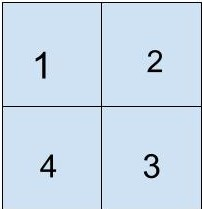0

# Ninja’s 3D structures

Difficulty: MEDIUM
Avg. time to solve
15 min
Success Rate
85%

Problem Statement
Suggest Edit

#### Note:

``````The bottom face of each shape counts toward its surface area.
``````

#### Example

``````Let say ‘N’ = 2 and ‘GRID’ = [ [1,2] , [ 4, 3] ]. The the grid will look like this
````````````Here, Ninja has kept 1 cube at ‘GRID’, 2 cubes at ‘GRID’, 4 cubes at ‘GRID’ and three cubes at ‘GRID’.

If we have to find the total surface area of this structure then, we will have to subtract the total surface area that has glued up.

After subtracting, the total surface area of the structure made by Ninja would be 34.
``````
##### Input Format:
``````The first line contains a single integer ‘T’ representing the number of test cases.

The first line of each test case will contain a single integer ‘N’ which denotes the grid’s size.

The next ‘N’ lines contain the ‘N’ space-separated integers which denote the value of ‘GIRD[i][j]’.
``````
##### Output Format:
``````For each test case, return the total surface area of the structure.
``````
##### Note:
``````You don’t need to print anything; It has already been taken care of. Just implement the given function.
``````
##### Constraints:
``````1 <= T <= 50
1 <= N <= 100
1 <= GRID[ i ][ j ] <= 50

Time limit: 1 sec
``````
##### Sample Input 1:
``````2
2
1 3
3 3
1
2
``````
##### Sample Output 1:
``````32
10
``````
##### Explanation of sample input 1:
``````In the first test case, there is 1 cube at “grid” , 3 cubes at “grid” ,“grid” , and   “grid”. So if we consider surface area of all cube towers independently then it would be
(6 * (1^2) ) + (3 * 6 * (1^2) ) + (3 * 6 * (1^2) ) +  (3 * 6 * (1^2) ) = 60.

But here, the cube at “grid[0,0]” is sharing the surface with cubes at “grid” and at “grid”. Similarly, other cubes also have surfaces glued together. After subtracting the glued area, the total surface area of the structure will be 32 units.

In the second test case, there are only 2 cubes towers placed together. Their surface area individually will be 2 * (6 * (1^2) ) = 12, but the topmost surface of the first cube and the bottom-most surface of the second cube is glued together and not will be considered in the total surface area, so the total surface area will be 2 * (6 * (1^2) ) - (2 * (1^2) ) = 10.
``````
##### Sample Input 2:
``````2
4
1 4 3 5
7 8 9 0
1 0 3 0
1 1 1 1
1
0
``````
##### Sample Output 2:
``````128
0
``````
##### Explanation of sample input 2:
``````In the second test case, there is no cube in the grid, so the surface area is 0.
``````Console